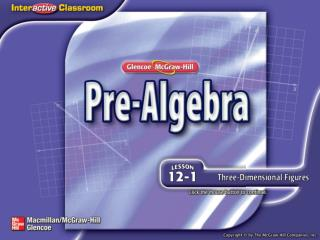DownloadDownload PresentationSplash Screen

# Splash Screen

Télécharger la présentation## Splash Screen

- - - - - - - - - - - - - - - - - - - - - - - - - - - E N D - - - - - - - - - - - - - - - - - - - - - - - - - - -
##### Presentation Transcript

1. Splash Screen

2. Five-Minute Check (over Chapter 11) Then/Now New Vocabulary Concept Summary: Polyhedrons Example 1: Identify Solids Example 2: Cross Section of a Cone Example 3: Describe and Draw Lesson Menu

3. ___ If ΔFOG ΔHAT, what segment is congruent to TH? ___ ___ ___ ___ A.FG B.GF C.OG D.GO 5-Minute Check 1

4. The vertices of figure WXYZ are W(2, 3), X(4, 5), Y(5, 1) and Z(1, –2). What are the coordinates after WXYZ is rotated 90˚ clockwise about the origin? A.W'(–2, 3), X'(–4, 5), Y'(–5, 1), Z'(–1, 2) B.W'(2, –3), X'(4, –5), Y'(5, –1), Z'(1, 2) C.W'(3, 2), X'(5, 4), Y'(–1, –5), Z'(2, 1) D.W'(3, –2), X'(5, –4), Y'(–1, –5), Z'(–2, –1) 5-Minute Check 2

5. Find the value of x. A. 135 B. 55 C. 45 D. 35 5-Minute Check 3

6. What is the area of a triangle with a base of 34 centimeters and a height of 19 centimeters? A. 323 cm2 B. 332 cm2 C. 644 cm2 D. 646 cm2 5-Minute Check 4

7. What is the circumference of a round table with a diameter of 25 inches? A. 75.8 in. B. 78.5 in. C. 151.6 in. D. 157 in. 5-Minute Check 5

8. Find the area of the figure. A. 215.1 mm2 B. 169.4 mm2 C. 158.5 mm2 D. 69.7 mm2 5-Minute Check 6

9. You have already modeled three-dimensional figures. (Lesson 12–1a) • Identify three-dimensional figures. • Describe and draw vertical, horizontal, and angled cross sections of three-dimensional figures. Then/Now

10. plane • base • solid • polyhedron • edge • vertex • face • prism • pyramid • cylinder • cone • cross section Vocabulary

11. Concept

12. edges: Identify Solids Identify the figure. Name the bases, faces, edges, and vertices. Answer: This figure has two parallelcongruent bases thatare rectangles, GHJK and LMNP, soit is arectangular prism. faces:GHJK, LMNP, GHML, HJNM, JKPN, GKPL vertices:G, H, J, K, L, M, N, P Example 1

13. A.rectangular pyramid with base: BCDE B. faces: ABC, ACD, ADE, AEB, BCDE C. D.vertices: B, C, D, E Identify the figure. Name the bases, faces, edges, and vertices. Which listing is not accurate? Example 1 CYP

14. Cross Section of a Cone Draw and describe the shape resulting from a vertical, angled, and horizontal cross section of a rectangular pyramid. Answer: Example 2

15. What is the shape of a horizontal cross section through the cylinder below? A. circle B. rectangle C. parabola D. triangle Example 2 CYP

16. Describe and Draw GIFT BOX A gift box is shown below. Draw the top view and side view. Then draw and describe the shape resulting from a vertical cross section of the figure. Answer: Example 3

17. Which is the top view of a triangular pyramid? A. B. C. D. Example 3 CYP

18. End of the Lesson